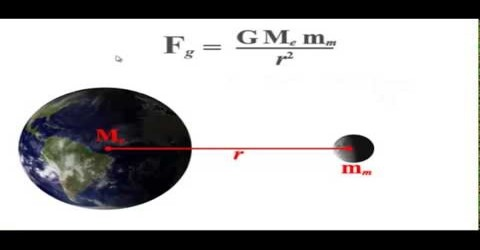# Application of Gravitation Law

Application of Gravitation Law

Newton’s gravitational law can only determine the force of attraction between two particles. But in reality each body has its own size and shape. When the distance between two bodies is very much larger than their sizes, only then we can ignore the sizes of the bodies and may consider them as particles. In this case the masses of the two bodies are considered to be concentrated at their centres and by applying Newton’s gravitational law we can calculate force of attraction. If the sizes of the extended bodies cannot be ignored then it is not easy to find the gravitational force of attraction.

Values of acceleration due to gravity varies at different places although mass remains constant. Consequently, weight of the body varies at different location. Due to the influence of gravitational force and variations of locations variations of gravitational potential and intensity along with the acceleration due to gravity occur.

By assuming total Mass of any uniform solid sphere or uniform spherical shell concentrated at their centres we can determine gravitational force of attraction on the particles of those spheres applying gravitational law.

Force of attraction does not act on particles inside the uniform spherical shell. In order to find the gravitational potential or intensity along with gravitational force on any point by applying law of gravitation the following phenomena may arise.

• The point may be inside the sphere
• The point may be on the sphere
• The pant may be outside the sphere.